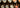# How to Select a Random Value from an Array?

javascript

27 Jun, 2022

Sometimes you have a list of values to choose from and you want to randomize it? It can be easily done with a line of code. This approach can be used in any programming language. Since I’m kinda comfortable with Javascript I’ve used JS code here. Please feel free to let me know your feedback and suggestions in the comments section. This article will help us understand how to select a random value from an array of values.

Getting a random number is easy. You just need to call Math.random() and it will produce a random decimal between 0 and 1 (exclusive). You just need to multiply it with the upper limit of your choice.

## How to use `Math.random()` and select a random value from an array?

The idea is simple, we just get a random index between 0 and array’s length and pick a value using that index. But the catch here is that Math.random() gives us a decimal with greater precision so we floor the random number to an integer.

The idea is simple, we just get a random index between 0 and array’s length and pick a value using that index. But the catch here is that Math.random() gives us a decimal with greater precision so we floor the random number to an integer.

Consider this code snippet,

```const arr = ['apple', 'orange', 'pineapple', 'banana', 'grapes']; // The code for picking a random value from an array is quite simple const randomValue = arr[Math.floor(Math. random() * arr.length)];```

Breaking the code

`Math.floor()` – Returns the largest integer less than or equal to a given number.

`Math.random()` – Returns a decimal between 0 (inclusive) and 1 (exclusive).

`Math.floor(Math. random() * arr.length)` – Return a random number between 0 and arr.length -1 (because Math.floor is used). We then use this random number as an index for picking an element from the array.

### Working Example

Here is a working CodePen to select a random value from an array. You can click the button to obtain a random value.

## Pro tip:

If you want a value to be repeated often or you wanted to give more weight to a particular value, repeat that value two or more times in the array. For example, if you want more `apple` returned while getting random values, use `apple` twice or thrice.

`const arr = ['apple', 'apple', 'apple', 'orange', 'pineapple', 'banana', 'grapes'];`

In this way, you will get more apples returned than other values. I hope this article helps you to get a random value from an array of values. More tutorials and content is coming up your way. Also, check out my previous articles on how to grow your online presence and how wordpress.org differs from wordpress.com.

Consider subscribing to the newsletter for more tips and hacks like this.
00:00
Can you stay a bit longer?